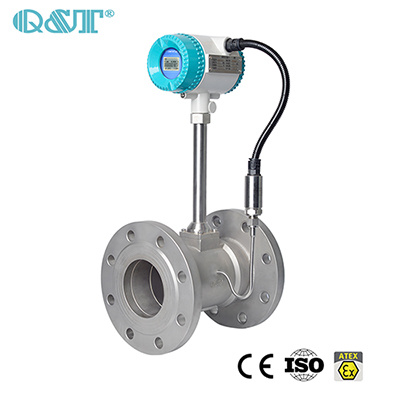Vortex flow meter working condition volume flow conversion method

2022-08-29
Vortex flow meter working condition volume flow conversion method

In the production process of the vortex flow meter, we need to determine an important parameter: flow parameters; the flow range of the vortex flow meter is basically fixed, and the selection is based on the working condition flow. When we know the standard conditions of our street flow meter, how can we calculate the working condition flow to make the caliber option.

The installation conditions of vortex flow meter below DN300 are: pipeline type, above DN300 are: plug-in type, which can be used to detect the flow of various liquid and gas media, not only can detect the volume flow of the medium, but also can detect the mass flow of the medium. It is very important to select the installation point correctly and install the flow meter correctly. If the installation is wrong, it will affect the measurement accuracy and even damage the vortex flow meter.

Vortex flow meter instrument coefficient: It has been debugged and tested before leaving the factory. The instrument constant K of each flow meter has been indicated on the nameplate and factory certificate. Its physical meaning is in the calibrated state (P=101.3kPa , t=20°C) The number of pulses output by the flow meter for every 1 liter of volume flow through the flow meter, the unit is 1/L. Due to the temperature change of the measuring medium, the geometric size of the measuring pipe and the vortex generating body changes (thermal expansion and cold contraction), the flow meter constant of the vortex flow meter needs to be corrected. The expression of the correction coefficient KT is: KT=1-4.8× 10-5×(t-20) In the formula: t──the temperature of the measuring medium, ℃.

Vortex flow meter working condition volume flow conversion method:

(1): Calculate the volume flow under the actual pipeline working state according to the flow range of the vortex flow meter set by the process:

The flow rate set by the process can be mass flow (kg/h), volume flow in working state (m3/h) or volume flow in standard state (N m3/h). Convert mass flow or volume flow in standard state into The method of volume flow in working state is as follows:

a. The vortex flow meter converts the maximum flow (upper range) Gmax (kg/h) of the mass flow into the volume flow Qmax (m3/h) in the working state, and the calculation formula is:

Qmax= Gmax×─ (m3/h)

In the formula: ρ──the density of the medium under the working conditions of the instrument (kg/m3)

b. Convert the maximum flow rate (upper limit of the range) Q0max (Nm3/h) in the standard state of the gas into the volume flow Qmax (m3/h) in the working state, and the calculation formula is:

Qmax= Q0max×──────×────── (m3/h)

In the formula: P——the gauge pressure of the gas under the working conditions of the instrument (MPa);

t——The temperature of the gas under the working conditions of the instrument (℃).

(2): Calculate the maximum frequency fmax of the vortex street according to the maximum volume flow Qmax (m3/h) in the working state:

fmax = ───×Qmax×K×KT (Hz)

In the formula: K——the meter coefficient (1/L), the K value is indicated on the meter nameplate;

KT——Temperature correction coefficient.share: